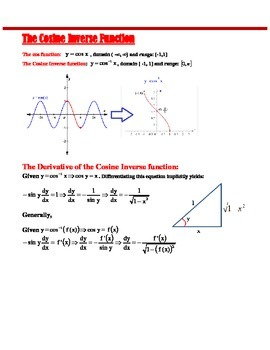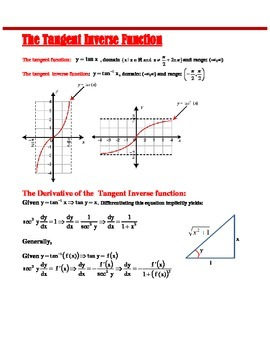# CALCULUS: DERIVATIVES OF INVERSE TRIG FUNCTIONSSubject
Resource Type
Product Rating
File Type

PDF (Acrobat) Document File

227 KB|8 pages
Share
Product Description
This lesson on how to derivatives of inverse trigonometric functions is intended for students enrolled in AP Calculus AB or BC, Calculus Honors, or College Calculus.

The idea about the derivatives of inverse trigonometric functions is quite difficult to grasp in the first level of calculus.

In this electronic document, I have provided a simple outline showing how the formulas are derived. The formulas are then generalized so that it is possible to find the derivative of any inverse trigonometric function composition.

The document discusses the following:

1. The derivative of the inverse sine function.
2. The derivative of the inverse sine function
3. The derivative of the inverse tangent function
4. The derivative of the inverse secant function

I have deliberately left the other inverse trigonometric functions because the 4 basic ones are sufficient enough to illustrate the idea. Teachers who teach AP Calculus or Calculus 1 in the university will find this document useful. Students also taking the subject can use the document.
Total Pages
8 pages